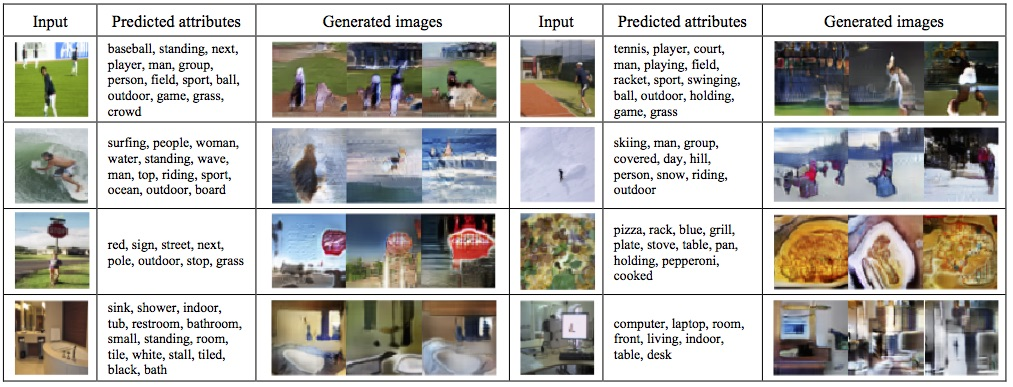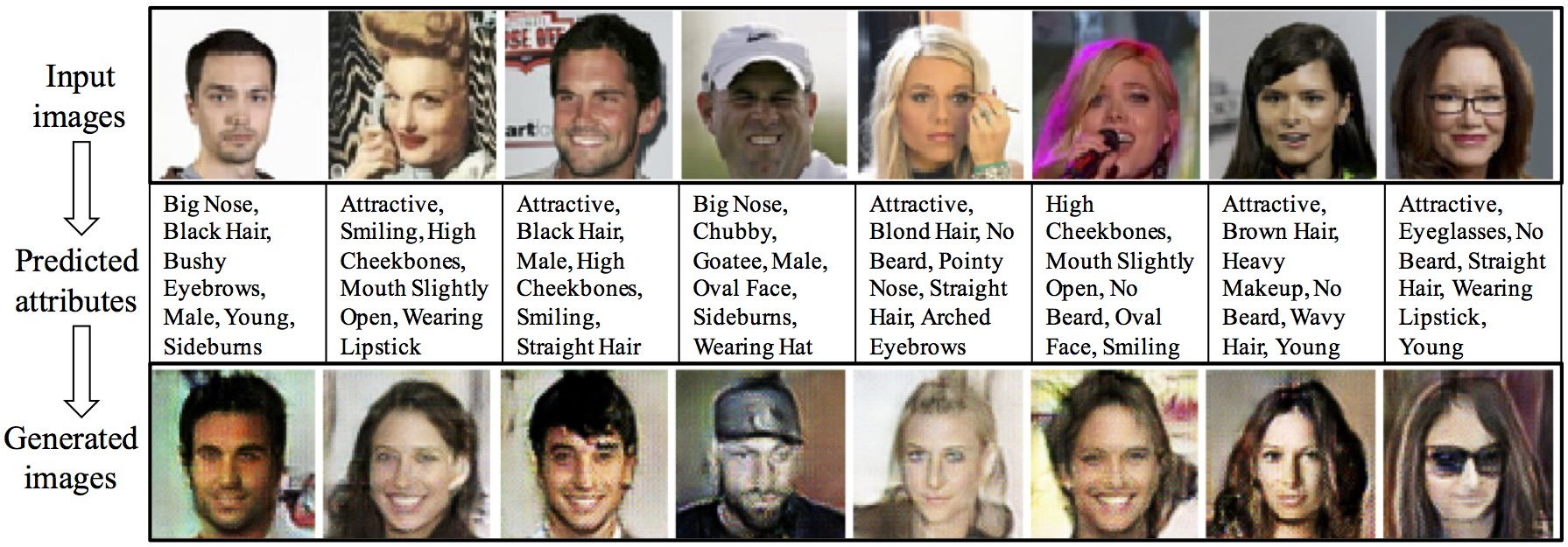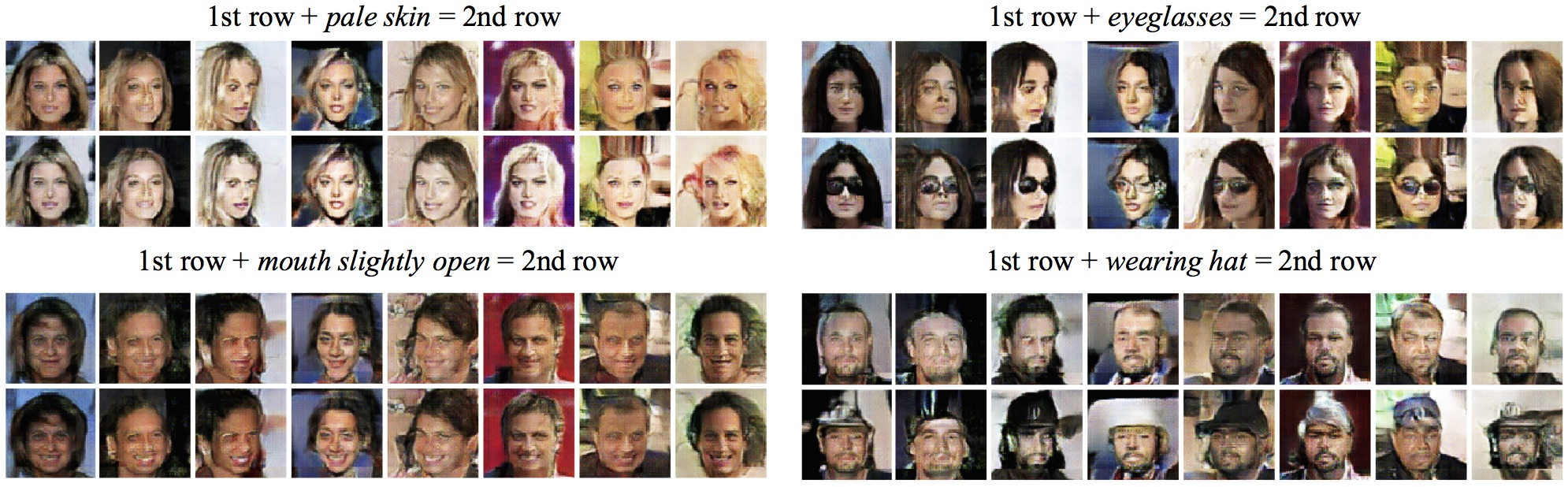LiqunChen0606/Triangle-GAN

implementation for NIPS paper Triangle Generative Adversarial Networks
Latest commit 405a0a3 Jan 23, 2018
Type Name Latest commit message Commit time
Failed to load latest commit information.demofiguressrc Jan 23, 2018README.md Oct 30, 2017

Triangle-GAN

This is an implemtation for NIPS paper: Triangle Generative Adversarial Networks

1. Experiments Settings:

1. Running environment:

tensorflow 1.1.0, python 2.7;

2. Dataset Format

For domain transfer and classification task, CelebA and MSCOCO dataset need to be in HDF5 format;

3. Resources

For domain transfer and classification task: If you want to re-run the CelebA experiment, the feature can be downloaded here: CelebA tag features

2. Basic Model:

Here's is our model: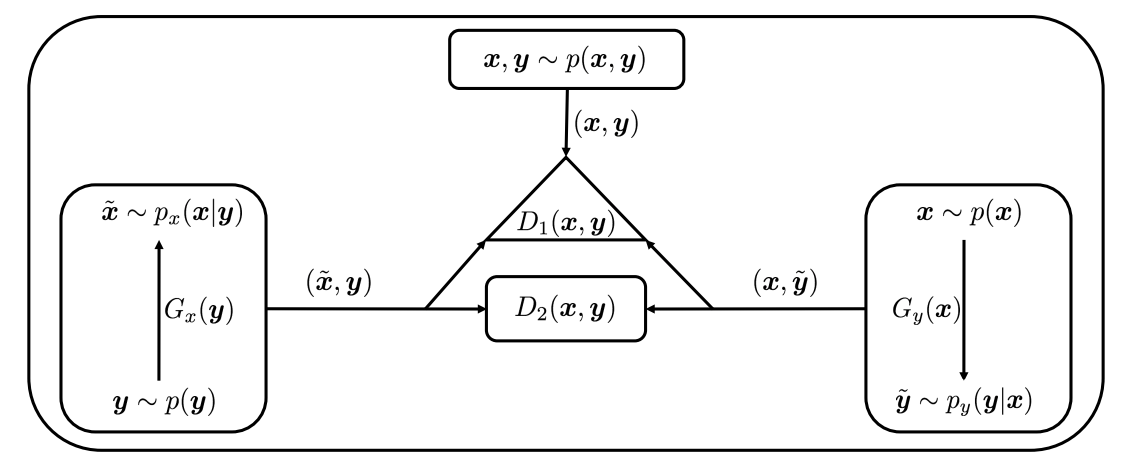The value function for TriGAN model: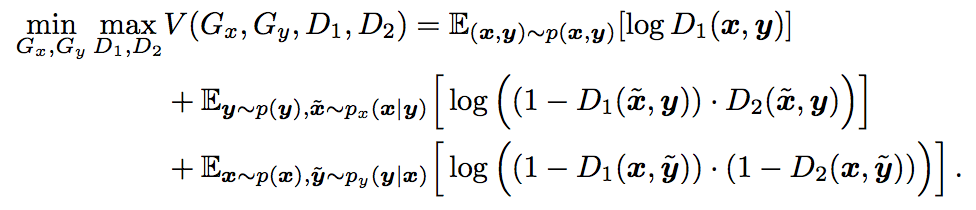The objective of $\Delta$-GAN is to match the three joint distributions: $p(x, y)$, $p_x(x, y)$ and $p_y(x, y)$. If this is achieved, we are ensured that we have learned a bidirectional mapping $p_x(x|y)$ and $p_y(y|x)$ that guarantees the generated fake data pairs $(\hat{x}, y)$ and $(x, \hat{y})$ are indistinguishable from the true data pairs $(x, y)$. In order to match the joint distributions, an adversarial game is played. Joint pairs are drawn from three distributions: $p(x, y)$, $p_x(x, y)$ or $p_y(x, y)$, and two discriminator networks are learned to discriminate among the three, while the two conditional generator networks are trained to fool the discriminators.

3. Compare with simplified Triple GAN: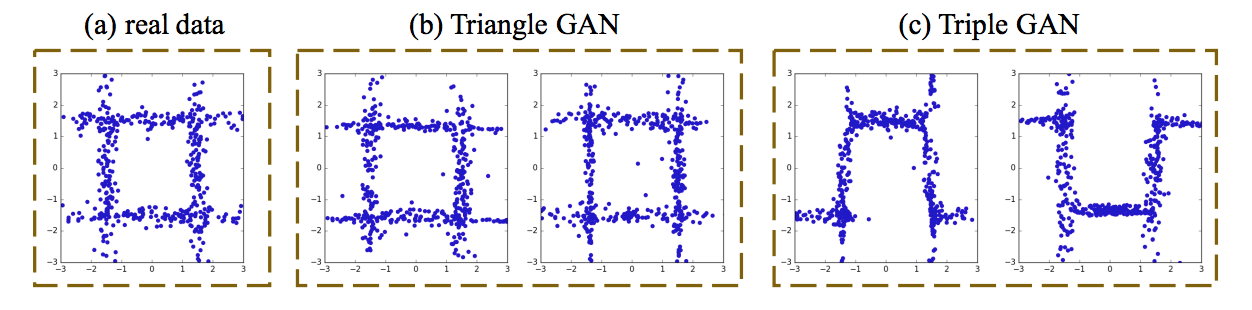figure (a): the joint distribution $p(x,y)$ of real data.

figure (b): $\Delta$-GAN left: the joint distribution $p_x(x,y)$; right: the joint distribution $p_y(x,y)$.

figure (c): Tirple GAN without regularization terms left: the joint distribution $p_x(x,y)$; right: the joint distribution $p_y(x,y)$.

4. Results

1. conditional generated CIFAR images: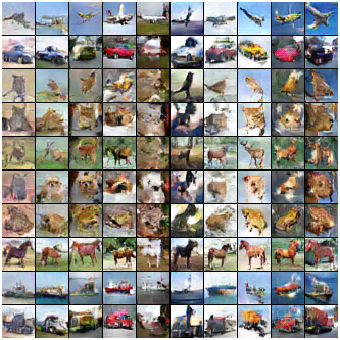2. MSCOCO:

1. generated COCO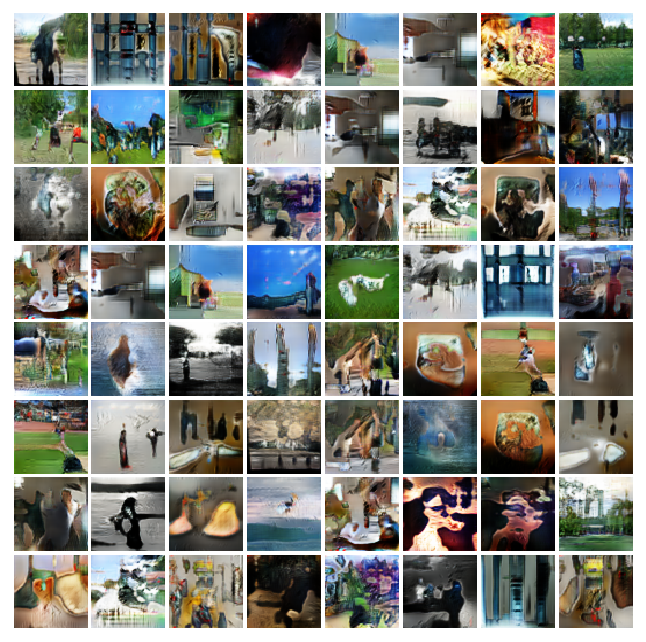2. COCO to attributes to COCO